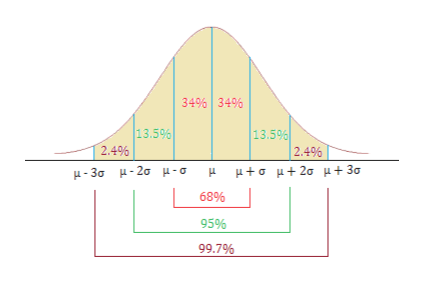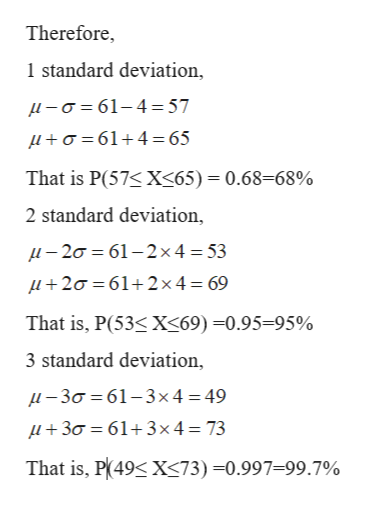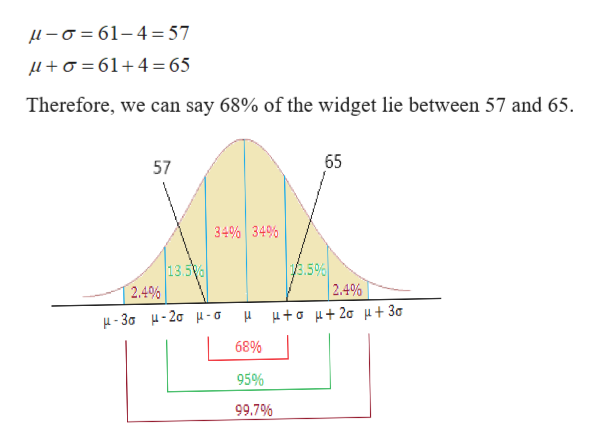# The Acme Company manufactures widgets. The distribution of widget weights is bell-shaped. The widget weights have a mean of 61 ounces and a standard deviation of 4 ounces.Use the Standard Deviation Rule, also known as the Empirical Rule (see image below). Do not use normalcdf on your calculator.Suggestion: sketch the distribution in order to answer these questions.a) 68% of the widget weights lie between andb) What percentage of the widget weights lie between 49 and 65 ounces? %c) What percentage of the widget weights lie below 69 ? %

Question
2 views

The Acme Company manufactures widgets. The distribution of widget weights is bell-shaped. The widget weights have a mean of 61 ounces and a standard deviation of 4 ounces.

Use the Standard Deviation Rule, also known as the Empirical Rule (see image below). Do not use normalcdf on your calculator.

Suggestion: sketch the distribution in order to answer these questions.

a) 68% of the widget weights lie between and

b) What percentage of the widget weights lie between 49 and 65 ounces? %

c) What percentage of the widget weights lie below 69 ? %

check_circle

Step 1

Given:

X: Widget weights

Mean = μ = 61

Standard deviation = σ = 4

Empirical rule tells us that within one standard deviation from mean 68% of data lie, within two standard deviations from mean 95% of data lie, and within 3 standard deviations from mean 99.7% of data lie as shown in figure.help_outlineImage Transcriptionclose34% 34% 13.5% 2.4% 13.5% 2.4% μ+σ μ+ 2σ μ+3 μ-3σ μ - 2σ μ- σ 68% 95% 99.7% fullscreen
Step 2help_outlineImage TranscriptioncloseTherefore, 1 standard deviation, L-61-4= 57 La614= 65 That is P(57< X<65)= 0.68=68% 2 standard deviation, -20 61-2x4 = 53 +20 612x 4 69 That is, P(53X<69) =0.95-95% 3 standard deviation, -30 61-3x4= 49 30 61+3x 4 = 73 That is, P/49 X<73) -0.997=99.7% fullscreen
Step 3

a)68% of the widget.

So, we can say 68% of the data lie...help_outlineImage Transcriptionclose-a61-4 57 a61 4 = 65 Therefore, we can say 68% of the widget lie between 57 and 65 65 57 34% 34% /3.5% 2.4% 13.5% 2.4% μμ+σ μ+2σ μ+ 3σ μ-36 μ- 2σμ-σ 68% 95% 99.7% fullscreen

### Want to see the full answer?

See Solution

#### Want to see this answer and more?

Solutions are written by subject experts who are available 24/7. Questions are typically answered within 1 hour.*

See Solution
*Response times may vary by subject and question.
Tagged in

### Statistics